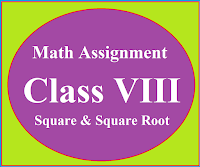### Math Assignment Class VIII | Square & Square RootMath Assignment  Class VIII | Square & Square Root Download or Print free  assignment with answer key  for   Class  8 Squares and  Square Roots.   Important and extra questions that cover all topics of square and square root and is useful and helpful for the students. Math Assignment  Class VIII | Square & Square Root LEVEL -1

## Math Assignment  Class XII  Ch - 04DETERMINANTS

Extra questions of chapter 04 Determinants class XII  with answers and  hints to the difficult questions, strictly according to the CBSE syllabus. Important and useful math. assignment for the students of class XII

## Strictly according to the CBSE Board

Question 1

Find the value of k for which matrix A is a singular matrix

Ans: 土 4

Question 2

If A is a square matrix of order 3 and |A| = -4, then find the value of |adj A|

Ans: 16

Question 3

,

and |A3| = 27, then find the value of α

Ans: √7

Question 4

, then find the value of x

Ans : x = 9

Question 5

then verify that |AB| = |A||B|

Solution Hint: |AB| = 77 and |A||B| = 7 x 11 = 77

Question 6

Ans: a2 + b2 + c2 + d2

Question 7

Ans: 0

Question 8

For what value of x, the matrix A is singular

Question 9

Find the area of triangle with vertices A(5, 4), B(-2, 4), C(2, -6)

Ans: 35 sq unit

Question 10

Using determinants show that the points (2, 3), (-1, -2) and (5, 8) are collinear

Solution Hint:

Find area of triangle by taking above given points as vertices.

If area of triangle = 0 then points are collinear.

Question 11

Using determinants find the value of k so that the points (k, 2 - 2k), (- k + 1, 2k), and (- 4 - k, 6 - 2k) may be collinear

Ans: k = -1, 1/2

Question 12
Using determinants, find the equation of line joining the points (3,1), and (9,3)

Ans: x - 3y = 0

Question 13

Find the value of k, if area of triangle is 4 square units whose vertices are (-2,0), (0,4), and (0, k)

Ans: K = 0, 8

Question 14

Find the value of |AB| if matrices A and B are given below

and

Ans: 0

Question 15

Find the value of x if matrix A is a singular matrix

Ans: x = -4

Question 16

Find the product :

Hence solve the following system of equations
x - y = 3, 2x + 3y + 4z = 17, y + 2z = 7

Ans: x = 2, y = -1, z = 4

Solution Hint
Let given matrices are A and C

Now find the product AC we get

Now given system of equations can be written as

AX = B ⇒ X =
A-1B
⇒ x = 2, y = -1, z = 4 is the required solution

Question 17

Find A-1  and hence solve following system of equations

3x - 4y + 2z = -1, 2x + 3y + 5z = 7, x + z = 2

Ans: x = 3, y = 2, z = -1

Solution Hint:

Find |A| we find |A| = -9 ⇒ A is invertible

Find cofactors of A and then find Adj. A we get

Find A-1  we get

Given system of equations can be written as AX = B
⇒ X= A-1B

⇒ x = 3, y = 2, z = -1

Question 18

Find A-1. Use A-1 to solve the system of equations.

2x – 3y + 5z = 11, 3x + 2y – 4z = - 5, x + y - 2z = - 3

Ans: x = 1, y = 2, z = 3

Solution Hint

Find the |A| we get |A| = -1

Solve: X = A-1B we get x = 1, y = 2, z = 3

Question 19

Show that the matrix A satisfies the equation A - 4A - 5I = O and hence find A-1

Ans:

Question 20

Find the matrix X for which

Ans

Question 21

Find  (AB)-1

Ans:
Question 22
Solve the following system of equations
x + y + z = 3, 2x - y + z = -1, 2x + y - 3z = -9
Ans:  x = -8/7,  y=10/7,  z=19/7

Question 23
Solve the following system of equations

where x, y, z ≠ 0
Ans: x = 2, y = 3, z = 5

Question 24

If A =,  B =   and X be a matrix such that  A = BX, then find X